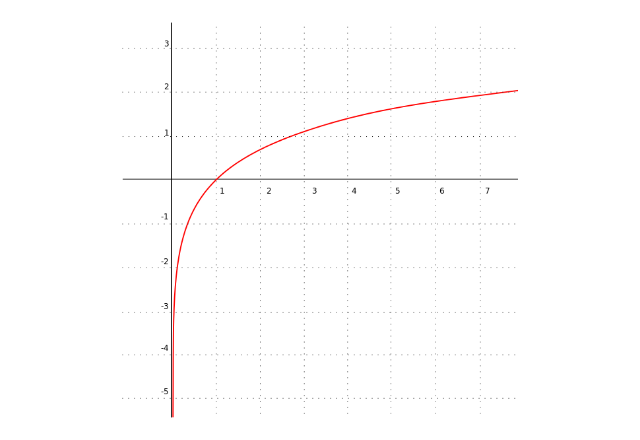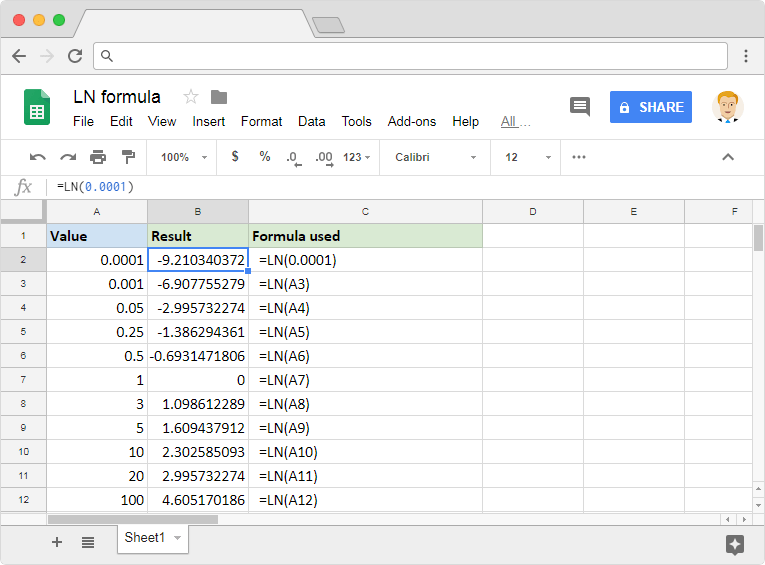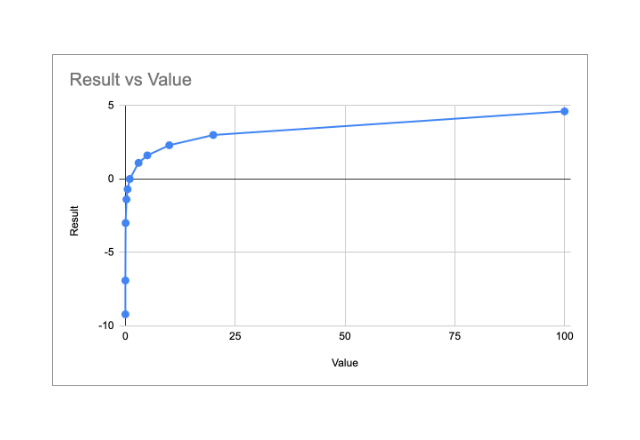# How to use the LN formula in Google SheetsSpreadsheets of today are comprehensive tools that help us with everyday calculations. Be it elementary or advanced, they got a bundle of mathematical functions to offer. The LN formula in Google Sheets helps us to calculate the natural logarithm of a value. It evaluates the logarithm value considering the Euler’s number (e) (a mathematical constant) for its base.

This function is abundantly used in the field including but not limited statistics, probability, and computing. Here’s how the function looks like when we plot it on the graph.### Syntax

LN(value)

• value – The value for which to calculate the logarithm, base e.

Please note that input for value parameter must be positive. If we tried to use it with a zero or negative value, it throws up a #NUM! error. That is because the logarithm is undefined for zero or negative numbers.

### Usage: LN formula in Google Sheets

It is now time that we practically apply the formula in Google Sheets application. And we may compare the graph with that of the output values. Please have a look at the following snapshot.Similar to most other Google Sheets formulas, the LN formula accepts direct numeric values. It also is capable of consuming references to the cells that contain numeric values. The first example has an input that is a direct numeric value. Whereas all other examples have cell references for its input.

Now let’s observe the output values the formula returned. If we made a quick comparison of the output values against those in the graph, it certainly looks like it is following the graph in the first image. Let us venture out a little bit more and try to plot these output values on a graph using Google Sheets charts. And this is how it looks like. For sure, this chart resembles the first graph.